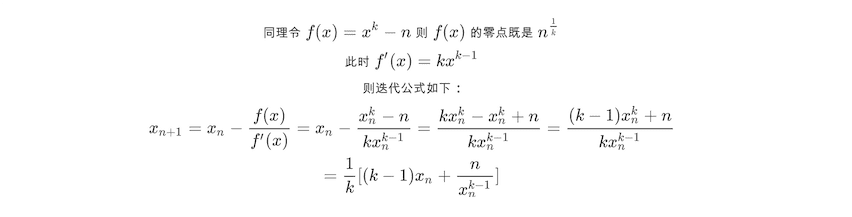# 编程与数学(三)：Newton‘s Method

9/27/2018

## Newton's Method## 应用

``````def int_sqrt(n):
"""
>>> int_sqrt(0)
0
>>> int_sqrt(1)
1
>>> int_sqrt(80)
8
>>> int_sqrt(81)
9
>>> int_sqrt(82)
9
"""
x_n = 1
x_n_plus_1 = (1 + n) / 2
#while int(x_n_plus_1) != int(x_n): 原来的错误做法，具体见评论
while abs(x_n_plus_1) != int(x_n):
x_n = x_n_plus_1
x_n_plus_1 = (x_n + n / x_n) / 2
return int(x_n_plus_1)``````

## 如果是开 k 次方呢？``````def int_sqrt_of(n, k=3):
"""
>>> int_sqrt_of(26, 3)
2
>>> int_sqrt_of(27, 3)
3
>>> int_sqrt_of(28, 3)
3
"""
x_n = 1
x_n_plus_1 = (k - 1 + n) / k
while abs(x_n_plus_1 - x_n) > 0.01:
x_n = x_n_plus_1
x_n_plus_1 = ((k - 1) * x_n + n / x_n ** (k - 1)) / k
return int(x_n_plus_1)``````